# Motion problem

From Levíc to Košíc go car at speed 81 km/h.
From Košíc to Levíc go another car at speed 69 km/h.

How many minutes before the meeting will be cars 27 km away?

Correct result:

t =  10.8 min

#### Solution:

$v_{1}=81 \ \text{km/h} \ \\ v_{2}=69 \ \text{km/h} \ \\ s=27 \ \text{km} \ \\ \ \\ v=v_{1}+v_{2}=81+69=150 \ \text{km/h} \ \\ t_{1}=\dfrac{ s }{ v }=\dfrac{ 27 }{ 150 }=\dfrac{ 9 }{ 50 }=0.18 \ \text{h} \ \\ t=t_{1} \rightarrow h=t_{1} \cdot \ 60 \ h=0.18 \cdot \ 60 \ h=10.8 \ h=\dfrac{ 54 }{ 5 }=10.8 \ \text{min}$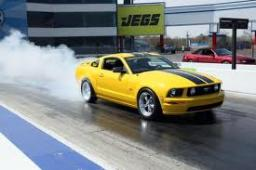Our examples were largely sent or created by pupils and students themselves. Therefore, we would be pleased if you could send us any errors you found, spelling mistakes, or rephasing the example. Thank you!

Leave us a comment of this math problem and its solution (i.e. if it is still somewhat unclear...):Be the first to comment!Tips to related online calculators
Need help calculate sum, simplify or multiply fractions? Try our fraction calculator.
Check out our ratio calculator.
Do you have a linear equation or system of equations and looking for its solution? Or do you have quadratic equation?
Do you want to convert length units?
Do you want to convert velocity (speed) units?
Do you want to convert time units like minutes to seconds?

## Next similar math problems:

• Two portsFrom port A on the river, the steamer started at an average speed of 12 km/h towards port B. Two hours later, another steamer departed from A at an average speed of 20 km/h. Both ships arrived in B at the same time. What is the distance between ports A an
• The touristThe tourist wanted to walk the route 16 km at a specific time. He, therefore, came out at the necessary constant speed. After a 4 km walk, however, he fell unplanned into the lake, where he almost drowned. It took him 20 minutes to get to the shore and re
• Water current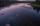John swims upstream. After a while, he passes the bottle, from that moment he floats for 20 minutes in the same direction. He then turns around and swims back, and from the first meeting with the bottle, he sails 2 kilometers before he reaches the bottle.
• FighterA military fighter flies at an altitude of 10 km. From the ground position, it was aimed at an altitude angle of 23° and 12 seconds later at an altitude angle of 27°. Calculate the speed of the fighter in km/h.
• Two shipsTwo ships sailed from Venice - a cargo speed of 10 knots and a ferry speed of 18 knots. At what time will the ferry catch up with the cargo ship if it sailed 10 hours later?
• Two trains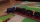Two trains departed from City A and City B against each other. They met after some time. The first train then took 9 hours to reach city B, and the second train took 4 hours to reach city A. In what proportion were the train speeds?
• The tentCalculate how much cover (without a floor) is used to make a tent that has the shape of a regular square pyramid. The edge of the base is 3 m long and the height of the tent is 2 m.
• Cyclists and walkersA group of tourists started at 8:00 at speed 4 km/h walk. At half-past ten, another group started on a bike and caught up with a group of tourists at 10:50. What was the average speed of cyclists?
• Average speed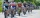The average speed of a pedestrian who walked 10 km was 5km/h, the average speed of a cyclist on the same track was 20km/h. In how many minutes did the route take more than a cyclist? Q
• Cyclist 12What is the average speed of a cycle traveling at 20 km in 60 minutes in km/h?
• Two trainsThe train runs at speed v1 = 72 km/h. The passenger, sitting in the train, observed that a train long l = 75m in 3 s passed on the other track in the opposite direction. Calculate the speed of this train.
• The shooter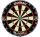The shooter heard the impact of the bullet on the target in one second after the shot. The bullet was moving at an average speed of 500 m/s. Calculate with speed of sound of 340 m/s. Determine the distance of the target.
• Runners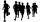If John has a running speed of 3.5miles per hour and Lucy has a speed of 5 miles per hour. If John starts running at 10:00 am and Lucy starts running at 10:30 am, at what time will they meet? (as soon as possible)
• Cheetah vs antelope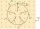When the cheetah began chasing the antelope, the distance between them was 120 meters. Although the antelope was running at 72km/h, the cheetah caught up with it in 12 seconds. What speed was the cheetah running?
• The tourist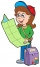The tourist walked a quarter of the way on the first day, a third of the rest on the second day, and 20 km on the last day. How many km did he walked in three days?
• The tourist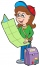The tourist has walked 3/4 of the route. The destination is 12.5 km away. How many kilometers did the route measure?
• Fast tourists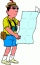If three tourists pass the route in 5 hours, how long will the same route take six equally fast tourists?